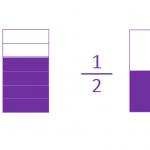Smartick is an advanced online program that teaches kids math and coding in only 15 min. a day

# I want to learn about: Number & Operations – Fractions

Content about fractions in elementary mathematics. Exercises, tutorials, problems, and teaching resources for fractions in elementary. Here you will find posts about fractions to make learning mathematics easier and more fun. ido generado sobre fracciones de matemáticas de primaria.

May05

## Half, Third, Fourth, Fifth in Math: Definition & CalculationIn this post, we are going to learn how to calculate a half, third, fourth, and fifth. These expressions are not just used for math problems, but also in daily life! Do you know what they are? Do you know how to calculate them? You are going to see how easy it is to calculate […]

Apr20

## Adding Fractions with Different Denominators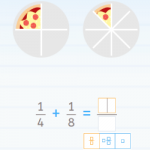We are going to begin this post about adding fractions with three very simple questions: 1. If you had one apple and were given two apples, how many apples do you have now? 2. If you had one apple and were given half an apple, how many apples do you have now? 3. If you […]

Mar16

## Equivalent Fractions on a Number Line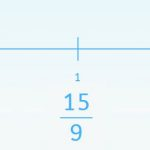Today we are going to learn how to solve activities related to the representation of fractions on a number line and the amplification and simplification of fractions. To do this we need to use equivalent fractions. It is easy to represent a fraction on a number line when it is divided into the same number […]

Nov18

## What Is a Fraction? Learn Everything There Is to Know!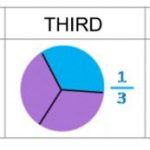On the Smartick blog we have dedicated many entries to explaining what a fraction is, what types there are, how to do addition, subtraction, multiplication and division with them, equivalent fractions and much more! In today’s post, we are going to organize these entries to make it easier for you to find the content you […]

Jul29

## Do You Know What an Equivalent Fraction Is?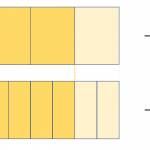Do you know what an equivalent fraction is? We have written about equivalent fractions many times on the blog: Equivalent Fractions Practice with Examples of Equivalent Fractions Examples of Equivalent Fractions Today, we are going to start by investigating the etymology, or meaning, of the word ”equivalent.” Let’s split the word in two: equi – […]

Apr29

## Multiplication of Fractions with an Area Model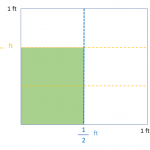In today’s post, we are going to see how to use an area model to solve a multiplication problem with fractions. This methodological strategy is very effective to show our students an operation that is very complicated to visualize. Teachers usually make an effort to support their explanations with manipulatives and visuals, because if they […]

Mar04

## Problems with Fractions and How to Solve ThemIn this post, we are going to give you some guidelines and advice for confronting problems with fractions. Problems with fractions, in no time! Fortywinklesburg is an imaginary kingdom where all of its inhabitants have a peculiar habit: they sleep for days without waking up. The Winklean’s, as they are called, sleep for so long […]

Nov19

## How to Add 3 Fractions with Different Denominators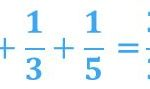On the Smartick blog, we have written a lot about fractions and the different operations we can do with them. In today’s blog post you can find much more information! Until now, we had not written about operations with more than 2 fractions. In this post, you are going to learn to add 3 fractions […]

Apr16

## Using the Number Line to Compare FractionsIn previous posts, we’ve learned how to place different numbers on the number line. Today we’re going to learn how to represent fractions on a number line. This is very useful when we need to compare them. Fractions with the same denominator As we know, it’s easy to compare fractions with the same denominator. In these cases, […]

Mar05

## Understanding Fractions: “If the Whole Is Made of 8 Parts, How Can I Have 11?”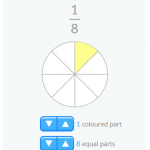We’ve written plenty about fractions in this blog. Recently, one of our readers asked us to explain the content of this post on classification of fractions in more detail. His comment was: “I’m sorry but I don’t understand improper fractions… I think it’s impossible to have 11 parts of something that’s divided into 8”. When we learn and […]

Feb05

## Adding and Subtracting Fractions: Why Do They Need the Same Denominator?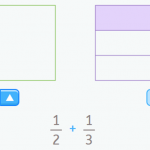Today we’re going to find out why, when we’re adding and subtracting fractions, they need to have the same denominator. If you didn’t already know, when we’re adding and subtracting fractions, they must be homogeneous. You can read more about homogeneous and heterogeneous fractions in this post. It’s really easy to understand using visual aids, which we’ll take […]